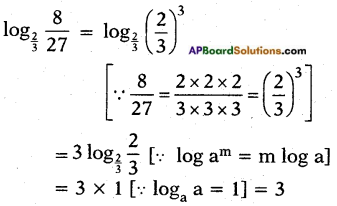# AP SSC 10th Class Maths Solutions Chapter 1 Real Numbers InText Questions

## AP State Syllabus SSC 10th Class Maths Solutions 1st Lesson Real Numbers InText Questions

AP State Board Syllabus AP SSC 10th Class Maths Textbook Solutions Chapter 1 Real Numbers InText Questions and Answers.

### 10th Class Maths 1st Lesson Real Numbers InText Questions and Answers

Do this

Question 1.
Find q and r for the following pairs of positive integers a and b, satisfying a = bq + r. (Page No. 3)
i) a = 13, b = 3
13 = 3 × 4 + 1
here q = 4 ; r = 1
ii) a = 8, b = 80
Take a = 80, b = 8
80 = 8 × 10 + 0 here q = 10 ; r = 0
iii) a = 125, b = 5
125 = 5 × 25 + 0
here q = 25 ; r = 0
iv) a = 132, b = 11
132 = 11 × 12 + 0
here q = 12 ; r = 0Question 2.
Find the HCF of the following by using Euclid division lemma,
i) 50 and 70 (Page No. 4)
For given two positive integers a > b;
there exists unique pair of integers q and r satisfying a = bq + r; 0≤r<b.
∴ 70 = 50 × 1 + 20
Here a = 70, b = 50, q = 1, r = 20.
Now consider 50, 20
50 = 20 × 2 + 10
Here a = 50, b = 20, q = 2, r = 10.
Now taking 20 and 10.
20 = 10 × 2 + 0
Here the remainder is zero.
∴ 10 is the HCF of 70 and 50.

ii) 96 and 72
96 = 72 × 1 + 24
72 = 24 × 3 + 0
∴ HCF = 24

iii) 300 and 550
550 = 300 × 1 + 250
300 = 250 × 1 + 50
250 = 50 × 5 + 0
∴ HCF = 50

iv) 1860 and 2015
2015 = 1860 × 1 + 155
1860 = 155 × 12 + 0
∴ HCF = 155Think & Discuss

Question 1.
From the above questions in ‘DO THIS’, what is the nature of q and r? (Page No. 3)
Given: a = bq + r
q > 0 and r lies in between 0 and b
i.e. q > 0 and 0 ≤ r < b

Question 2.
Can you find the HCF of 1.2 and 0.12? Justify your answer. (Page No. 4)
Given: 1.2 and 0.12
we have 1.2 = $$\frac{12}{10}$$ = $$\frac{120}{100}$$
0.12 = $$\frac{12}{100}$$
Now considering the numerators 12 and 120, their HCF is 12.
∴ HCF of 1.2 and 0.12 is $$\frac{12}{100}$$ = 0.12
i.e., if x is a factor of y then x is the HCF of x and y.

Question 3.
If r = 0, then what is the relationship between a, b and q in a = bq + r of Euclid divison lemma? (Page No. 6)
Given: r = 0 in a = bq + r then a = bq
i.e., b divides a completely.
i.e., b is a factor of a.Do this

Question 1.
Express 2310 as a product of prime factors. Also see how your friends have factorized the number. Have they done it as you ? Verify your final product with your friend’s result. Try this for 3 or 4 more numbers. What do you conclude? (Page No. 7)
Given: 2310
2310 = 2 × 1155
= 2 × 3 × 385
= 2 × 3 × 5 × 77
2310 = 2 × 3 × 5 × 7 × 11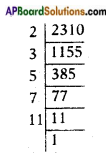We notice that this prime factorization is unique.
And also notice that prime factorization of any number is unique i.e., every composite number can be expressed as a product of primes and this factorization is unique.
E.g: 144 = 2 × 72
= 2 × 2 × 36
= 2 × 2 × 2 × 18
= 2 × 2 × 2 × 2 × 9
= 2 × 2 × 2 × 2 × 3 × 3
= 24 × 32
320 = 2 × 160
= 2 × 2 × 80
= 2 × 2 × 2 × 40
= 2 × 2 × 2 × 2 × 20
= 2 × 2 × 2 × 2 × 2 × 10
= 2 × 2 × 2 × 2 × 2 × 2 × 5
= 26 × 5
125 = 5 × 25
= 5 × 5 × 5
= 53

Question 2.
Find the HCF and LCM of the following given pairs of numbers by prime factorization, (Page No. 8)
i) 120, 90
We have 120 = 2 × 2 × 2 × 3 × 5
= 23 × 3 × 5
90 = 2 × 3 × 3 × 5
= 2 × 32 × 5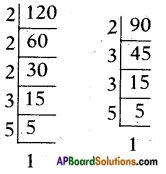∴ HCF = 2 × 3 × 5 = 30
LCM = 2<sup>3</sup> × 3<sup>2</sup> × 5 = 360

ii) 50, 60
We have
50 = 2 × 5 × 5 = 2 × 52
60 = 2 × 2 × 3 × 5 = 22 × 3 × 5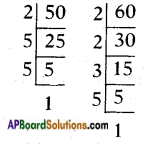∴ HCF = 2 × 5 = 10
LCM = 22 × 3 × 52 = 300

iii) 37, 49
We have
37 = 1 × 37
49 = 7 × 7 = 72
∴ HCF = 1
LCM = 37 × 72
Note: H.C.F. of two relatively prime numbers is 1 and LCM is equal to product of the numbers.Try this

Question 1.
Show that 3n × 4m cannot end with the digit 0 or 5 for any natural numbers ‘n’ and’m’. (Page No. 8)
Given number is 34 × 4m.
So the prime factors to it are 3 and 2 only.
I: but if a number want to be end with zero it should have 2 and 5 as its prime factors, but the given hasn’t ‘5’ as its prime factor.
So it cannot be end with zero.
II : now if a number went to be end with 5 it should have ‘5’ as its one of prime factors. But given 3n × 4m do not have 5 as a factor.
So it cannot be end with 5.
Hence proved.

Do this

Question 1.
Write the following terminating decimals in the form of p/q, q ≠ 0 and p, q are co-primes.
i) 15.265
ii) 0.1255
iii) 0.4
iv) 23.34
v) 1215.8
What can you conclude about the denominators through this process? (Page No. 10)
i) 15.265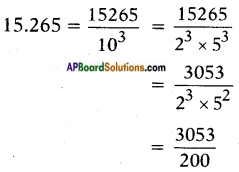ii) 0.1255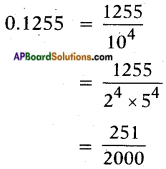iii) 0.4
0.4 = $$\frac{4}{10}$$ = $$\frac{2}{5}$$
iv) 23.34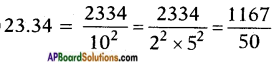v) 1215.8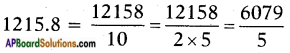Two and five are the factors for the denominator.Question 2.
Write the following rational numbers in the form of p/q, where q is of the form 2n.5m where n, m are non-negative integers and then write the numbers in their decimal form. (Page No. 11)
i) $$\frac{3}{4}$$
ii) $$\frac{7}{25}$$
iii) $$\frac{51}{64}$$
iv) $$\frac{14}{25}$$
v) $$\frac{80}{100}$$
i) $$\frac{3}{4}$$
$$\frac{3}{4}$$ = $$\frac{3}{2 \times 2}$$ = $$\frac{3}{2^{2}}$$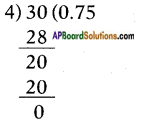Decimal form of $$\frac{3}{4}$$ = 0.75

ii) $$\frac{7}{25}$$
$$\frac{7}{25}$$ = $$\frac{7}{5 \times 5}$$ = $$\frac{7}{5^{2}}$$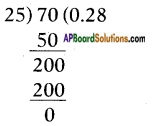Decimal form of $$\frac{7}{25}$$ = 0.28

iii) $$\frac{51}{64}$$
$$\frac{51}{64}$$ = $$\frac{51}{2^{6}}$$
[∵ 64 = 2 × 32
= 22 × 16
= 23 × 8
= 24 × 4 = 25 × 2 = 26]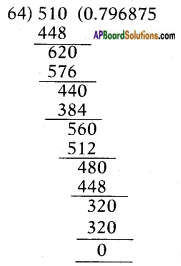Decimal form of $$\frac{51}{64}$$ = 0.796875

iv) $$\frac{14}{25}$$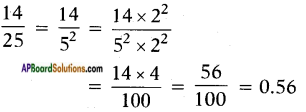v) $$\frac{80}{100}$$
$$\frac{80}{100}$$ = $$\frac{80}{2^{2} \times 5^{2}}$$ = $$\frac{80}{10^{2}}$$ = 0.80Question 3.
Write the following rational numbers as decimal form and find out the block of repeating digits in the quotient. (Page No. 11)
i) $$\frac{1}{3}$$
ii) $$\frac{2}{7}$$
iii) $$\frac{5}{11}$$
iv) $$\frac{10}{13}$$
i) $$\frac{1}{3}$$
$$\frac{1}{3}$$ = 0.3333…. = $$0 . \overline{3}$$
Block of digits, repeating in the quotient = period = 3.

ii) $$\frac{2}{7}$$
Decimal form of $$\frac{2}{7}$$ = 0.285714….
Repeating part/period = 285714
∴ $$\frac{2}{7}$$ = $$0 . \overline{285714}$$

iii) $$\frac{5}{11}$$
Period = 45
Decimal form of $$\frac{5}{11}$$ = 0.454545.
= $$0 . \overline{45}$$

iv) $$\frac{10}{13}$$
Decimal form of $$\frac{10}{13}$$ = 0.769230.
= $$0 . \overline{769230}$$
Period = 769230

Do this

Question 1.
Verify the statement proved above for p = 2, p = 5 and for a2 = 1, 4, 9, 25, 36, 49, 64 and 81. (Page No. 14)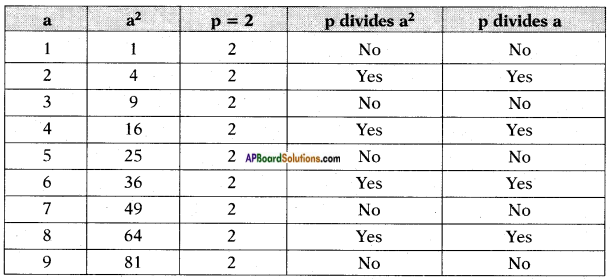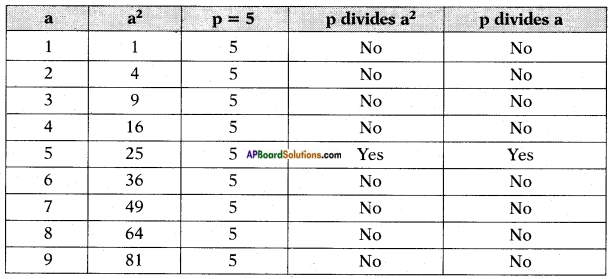From the above we can conclude that if a prime number ‘p’ divides a2, then it also divides a.Think and Discuss

Question 1.
Write the nature of y, a and x in y = ax. Can you determine the value of x for a given y? Justify your answer. (Page No. 17)
y = ax here a ≠ 0
We can determine the value of ‘x’ for a given y.
for example y = 5, a = 2
We cannot express y = ax for y = 5, a = 2 and for y = 7, a = 3, we cannot express seven (7) as a power of 3.

Question 2.
You know that 21 = 2, 41 = 4, 81 = 8 and 101 = 10. What do you notice about the values of log2 2, log4 4, log8 8 and log10 10? What can you generalise from this?  (Page No. 18)
From the graph log2 2 = log4 4 = log8 8 = log10 10 = 1
We conclude that loga a = 1 where a is a natural number.

Question 3.
Does log10 0 exist? (Page No. 18)
No, log10 0 doesn’t exist, i.e ax ≠ 0 ∀ a, x ∈ N.

Question 4.
We know that, if 7 = 2x then x = log2 7. Then what is the value of $$2^{\log _{2} 7}$$? Justify your answer. Generalise the above by taking some more examples for $$\mathbf{a}^{\log _{\mathrm{a}} \mathbf{N}}$$. (Page No. 21)
We know that if 7 = 2x then x = log2 7
We want to find the value of $$2^{\log _{2} 7}$$;
Now put log2 7 = x in the given
∴ $$2^{\log _{2} 7}$$ = 2x = 7 (given)
∴ $$2^{\log _{2} 7}$$ = 7
Thus $$\mathbf{a}^{\log _{\mathrm{a}} \mathbf{N}}$$ = N

a) $$3^{\log _{3} 8}$$
If x = $$3^{\log _{3} 8}$$ then
log3 x = log3 8
⇒ x = 8

b) $$5^{\log _{5} 10}$$
If y = $$5^{\log _{5} 10}$$
then log5 y = log5 10
⇒ y = 10

Do this

Question 1.
Write the powers to which the bases to be raised in the following.  (Page No. 18)
i) 64 = 2x
64 = 2x
We know that
64 = 2 × 32
= 2 × 2 × 16
= 2 × 2 × 2 × 8
= 2 × 2 × 2 × 2 × 4
= 2 × 2 × 2 × 2 × 2 × 2
64 = 26
⇒ x = 6ii) 100 = 5b
Here also 100 cannot be written as any power of 5.
i.e., there exists no integer for b such that 5b = 100

iii) $$\frac{1}{81}$$ = 3c
We know that 81 = 3 x 27
= 3 × 3 × 9
= 3 × 3 × 3 × 3
= 34
∴ $$\frac{1}{81}$$ = 3-4   [∵ a-m = $$\frac{1}{\mathrm{a}^{\mathrm{m}}}$$]
∴ c = – 4

iv) 100 = 10z
100 = 102
z = 2

v) $$\frac{1}{256}$$ = 4a
We know that 256 = 4 × 64
= 4 × 4 × 16
= 4 × 4 × 4 × 4
∴ $$\frac{1}{256}$$ = 4-4
∴ a = – 4

Question 2.
Express the logarithms of the following into sum of the logarithms.   (Page No. 19)
i) 35 × 46
log xy = log x + log y
log1035 × 46 = log1035 + log1046

ii) 235 × 437
log10235 × 437 = log10235 + log10437   [∵ log xy = log x + log y]

iii) 2437 × 3568
log10 2437 × 3568 = log102437 + log103568   [∵ log xy = log x + log y]

Question 3.
Express the logarithms of the follow¬ing into difference of the logarithms.   (Page No. 20)
i) $$\frac{23}{34}$$
log10 = $$\frac{23}{34}$$ = log10 23 – log10 34
[∵ log $$\frac{x}{y}$$ = log x – log y]

ii) $$\frac{373}{275}$$
log10 = $$\frac{373}{275}$$ = log10 373 – log10 275
[∵ log $$\frac{x}{y}$$ = log x – log y]

iii) $$\frac{4525}{3734}$$
log10 = $$\frac{4525}{3734}$$ = log10 4525 – log10 3734
[∵ log $$\frac{x}{y}$$ = log x – log y]

iv) $$\frac{5055}{3303}$$
log10 = $$\frac{5055}{3303}$$ = log10 5055 – log10 3303
[∵ log $$\frac{x}{y}$$ = log x – log y]Question 4.
By using the formula logaxn = n loga x, convert the following.   (Page No. 21)
i) log2 725
log2 725 = 25 log2 7

ii) log5 850
log5 850 = 50 log5 8 = 50 log5 23
= 3 × 50 log52 = 150 log52

iii) log 523
log 523 = 23 log 5

iv) log 1024
log 1024 = log 210 [∵ 1024 = 210]
= 10 log 2

Try this

Question 1.
Write the following relation in exponential form and find the values of respective variables.   (Page No. 18)
i) log232 = x
log232 = x
⇒ log225 = x     [∵ 32 = 25]
⇒ 5 log22 = x     [∵ log am = m log a]
⇒ 5 × 1 = x      [∵ loga a = 1]
∴ x = 5

ii) log5625 = y
log5625 = y
⇒ log54 = y    [∵ 625 = 54]
⇒ 4 log5 5 = y     [∵ log am = m log a]
⇒ 4 × 1 = y     [∵ loga a = 1]
∴ y = 4iii) log1010000 = z
log1010000 = z
=> log10104 = z     [∵ 10000 = 10 × 10 × 10 × 10 = 104]
=> 4 log1010 = z     [∵ log am = m log a]
=> 4 × 1 = z     [∵ loga a = 1]
∴ z = 4

iv) $$\log _{7} \frac{1}{343}$$ = -a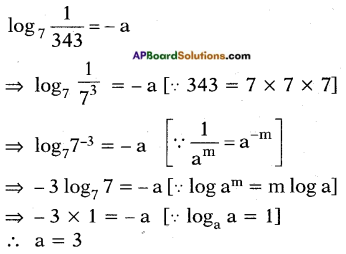Question 2.
i) Find the value of log232. (Page No. 21)
log2 32 = log2 25
[∵ 32 = 2 × 2 × 2 × 2 × 2 = 25]
= 5 log2 2 [∵ log am = m log a]
= 5 × 1 [∵ loga a = 1]
= 5

ii) Find the value of logc √c.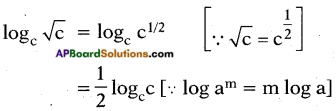= $$\frac{1}{2}$$ × 1 [∵ loga a = 1]
= $$\frac{1}{2}$$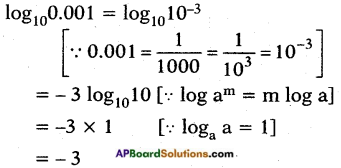iv) Find the value of $$\log _{\frac{2}{3}} \frac{8}{27}$$